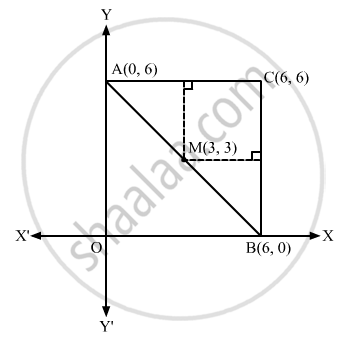Advertisement Remove all ads

# The Vertices of a Triangle Are (6, 0), (0, 6) and (6, 6). the Distance Between Its Circumcentre and Centroid is - Mathematics

MCQ

The vertices of a triangle are (6, 0), (0, 6) and (6, 6). The distance between its circumcentre and centroid is

#### Options

• $2\sqrt{2}$

• 2

• $\sqrt{2}$

• 1

Advertisement Remove all ads

#### Solution

$\sqrt{2}$ Let A(0, 6), B(6, 0) and C(6, 6) be the vertices of the given triangle.$\text { Centroid of } \bigtriangleup \text { ABC } = \left( \frac{0 + 6 + 6}{3}, \frac{6 + 0 + 6}{3} \right)$

$= \left( 4, 4 \right)$

$\text { Coordinates of N } = \left( \frac{6 + 6}{2}, \frac{6 + 0}{2} \right)$

$= \left( 6, 3 \right)$

$\text { Coordinates of P } = \left( \frac{0 + 6}{2}, \frac{6 + 6}{2} \right)$

$= \left( 3, 6 \right)$

Equation of MN is y = 3

Equation of MP is x = 3

As , we know that circumcentre of a triangle is the intersection of the perpendicular

bisectors of any two sides .

Therefore, coordinates of circumcentre is (3, 3)

Thus, the coordinates of the circumcentre are (3, 3) and the centroid of the triangle is (4,4).
Let d be the distance between the circumcentre and the centroid.

$\therefore d = \sqrt{\left( 4 - 3 \right)^2 + \left( 4 - 3 \right)^2} = \sqrt{2}$

Is there an error in this question or solution?
Advertisement Remove all ads

#### APPEARS IN

RD Sharma Class 11 Mathematics Textbook
Chapter 23 The straight lines
Q 31 | Page 135
Advertisement Remove all ads

#### Video TutorialsVIEW ALL 

Advertisement Remove all ads
Share
Notifications

View all notifications

Forgot password?